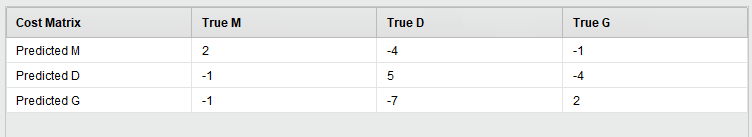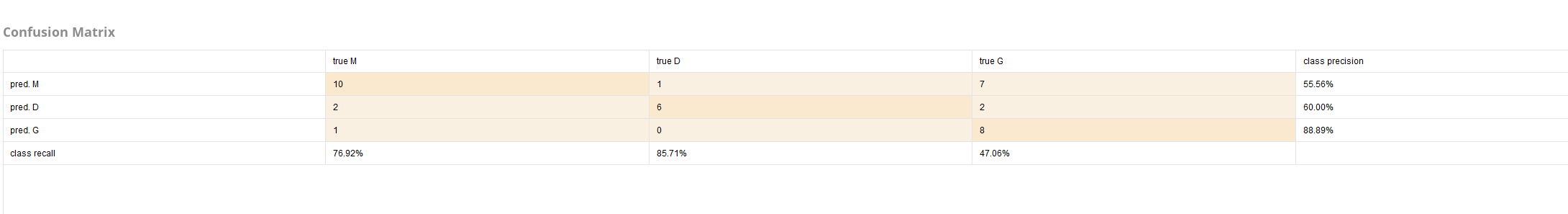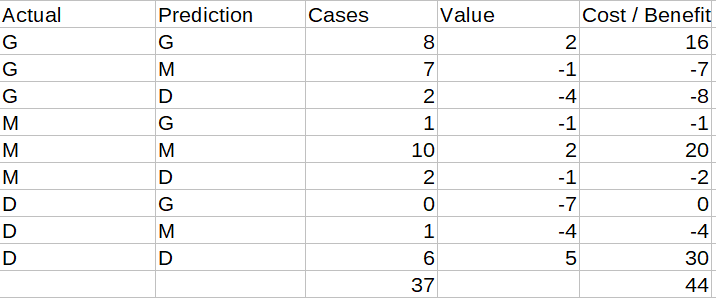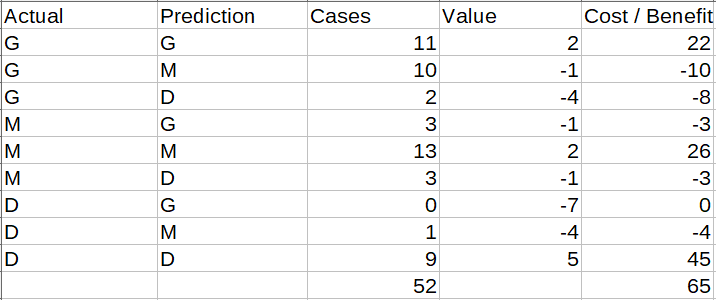# AutoModel Gains

Member Posts: 6Newbie
Hello, I am currently trying to understand the calculation of gains in Auto Model and can't come up with a solution. I have read the following post,

which states that the gain is calculated based on the predciton table, but this could be changed in a future update. I have now tried to calculate my gain based on the prediction table as well as on the confusion matrix, both unfortunately without success. I therefore have 3 on the subject.

1. Where is the error in my calculation the gain should be 63. But based on the matrix I get 44 and based on the table 65.

Matrix:Table:The Table is also attached.

2. Why do the matrix and the table contain a different number of attributes?

3. Is it possible to find the operator that calculates the gains outside of Automodel? I assumed that it is Performance(Costs) operator, but the results are different.